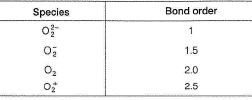Courses

# Retro (Past 13 Years) JEE Main (Chemical Bonding)

## 37 Questions MCQ Test Chemistry for JEE Advanced | Retro (Past 13 Years) JEE Main (Chemical Bonding)

Description
This mock test of Retro (Past 13 Years) JEE Main (Chemical Bonding) for JEE helps you for every JEE entrance exam. This contains 37 Multiple Choice Questions for JEE Retro (Past 13 Years) JEE Main (Chemical Bonding) (mcq) to study with solutions a complete question bank. The solved questions answers in this Retro (Past 13 Years) JEE Main (Chemical Bonding) quiz give you a good mix of easy questions and tough questions. JEE students definitely take this Retro (Past 13 Years) JEE Main (Chemical Bonding) exercise for a better result in the exam. You can find other Retro (Past 13 Years) JEE Main (Chemical Bonding) extra questions, long questions & short questions for JEE on EduRev as well by searching above.
QUESTION: 1

### Retro JEE Main  (Compilation of Last 13 Years  Questions) IIT JEE (Main & Advanced) past year questions with solutions & answer key Retro IIT JEE (Main and Advanced) have questions from previous/past (10 to 13 years) papers of IIT JEE (Main and Advanced) with solutions, The following set of questions contain previous/past (10 to  13 years) questions of IIT JEE (Main and Advanced) of Chapter "Chemical Bonding" with solutions    Q. The correct statement for the molecule Csl3 is (JEE Main 2014)

Solution: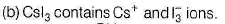QUESTION: 2

### For which of the following molecules significant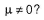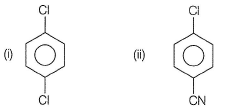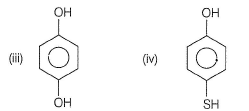Solution: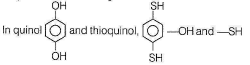groups do not cancel their dipole moments and hence exists as different conformations.

*Multiple options can be correct
QUESTION: 3

### Which one of the following molecules is expected to exhibit diamagnetic behaviour? (JEE Main 2013)

Solution: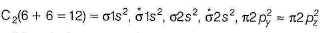Since, all the electrons are paired, it is a diamagnetic specie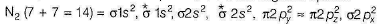It is also a diamagnetic specie because of the absence of un paired electrons.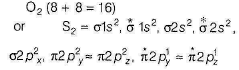Since, O2 or S2 contain 2 unpaired electrons, therefore they are paramagnetic.

QUESTION: 4

In which of the following pairs of molecules/ions both the species are not likely to exist?

(JEE Main 2013)

Solution:

Species having zero or negative bond order do not exist.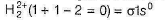Bond order = 0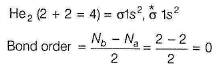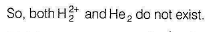QUESTION: 5

Stability of the species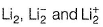increases in the order of

(JEE Main 2013)

Solution: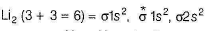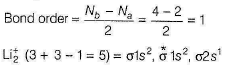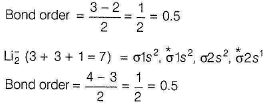Stability order is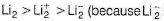have more number of electrons in antibonding orbitals which destabilises the species).

QUESTION: 6

The molecule having smallest bond angle is

(AIEEE 2012)

Solution: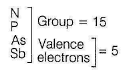MCl3 has sp3 hybridise  M-element with one lone pair.
Lone-pair and bond pair repulsion decreases bond angle. However, the bond pairs of electrons are much farther away from the central atom than they are in NCI3. Thus, lone pair causes even greater distortion in PCI3, AsCI3 and SbCI3.

Thus, bond angle decreases from NCI3 (maximum) to SbCI3 (minimum).

QUESTION: 7

In which of the following pairs, the two species  are not isostructural?

(AIEEE 2012)

Solution: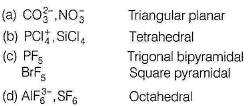QUESTION: 8

Among the following, the maximum covalent character is shown by the compound

(AIEEE 2011)

Solution:

Covalent character is favoured by Fajans ’ rule.
(i) Larger the charge on the ions,
(ii) Smaller the size of cations,
(iii) Larger the size of anions,
(iv) Cation with 18-electrons structure (e.g.Cu+),
then larger the polarising power and the covalent character is favoured.
In all the given compounds , anion is same (Cl-), hence polarising power is decided by size and charge of cation.
Al3+ with maximum charge and smallest size has maximum polarising power hence, AICI3 is maximum covalent.

QUESTION: 9

The hybridisation of orbitals of N atom in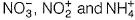Solution:

Count σ -bond , lone pairs and unpaired electrons or count number of atoms directly attached, lone pairs and unpaired electrons.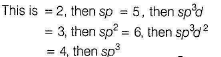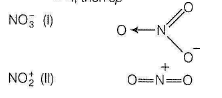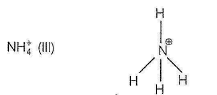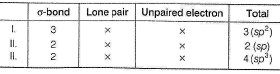QUESTION: 10

The structure of IF7 is

(AIEEE 2011)

Solution:

IF7 Outer orbital diagram of I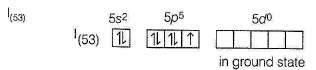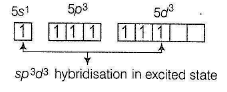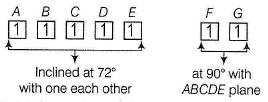Seven sp3d3 hybrid orbitalsforming s-bonds with F-atoms,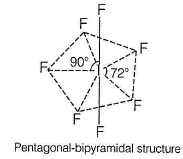QUESTION: 11

Which of the following has maximum number of lone pairs associated with Xe?

(AIEEE 2011)

Solution: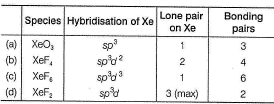QUESTION: 12

The number of types of bonds between two carbon atoms in calcium carbide is

(AIEEE 2011)

Solution: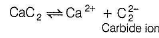In carbide ion, two carbon atoms are joined by triple bond (It is isoelectronic with N2)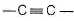with two π and one σ-bonds.

QUESTION: 13

Using MO theory, predict which of the following species has the shortest bond length?

(AIEEE 2009)

Solution:

Higher the bo nd order, shorter is the bond length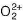has the shortest bond length (BO = 3).
Bond order of remaining species are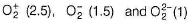QUESTION: 14

Which one of the following constitutes a group of the isoelectronic species?

(AIEEE 2008)

Solution:

Isoelectronic species have same number of electron,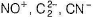and N2 all have 14 electrons.

QUESTION: 15

Which one of the following pairs of species have the same bond order?

(AIEEE 2008)

Solution:

CN- and NO+ both have same number of electrons and same bond order (3)

QUESTION: 16

Which of the following species exhibits diamagnetic behaviour?

(AIEEE 2007)

Solution:

The correct option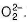This species has 18 electrons, which are filled in such a way that all molecular orbitals are fully filled, so diamagnetic.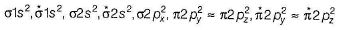QUESTION: 17

In which of the following ionisation processes, the bond order has increased and the magnetic behaviour has changed?

Solution: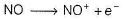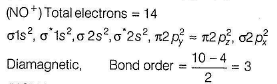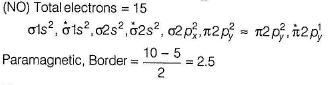Electron is taken away from non-bonding molecular orbital, thats why bond order increases.

QUESTION: 18

Which of the following hydrogen bond is the strongest?

(AIEEE 2007)

Solution:

Since, H - bond is the electrostatic force of attraction between H -atom and electronegative element. Thus, fluorine being the most electronegative element forms stronger H -bonds. On the other hand, H-—F possesses larger electronegative difference than O—H.
Therefore, H - F molecule is more polarised than O—H, due to which H -atom of H—F bears larger amount of partial charge than that of O—H. This suggests H - atom of H—F attracts more strongly F -atom than that of O—H.
Hence, H—F forms more stronger H - bonds with fluorine than that of O—H.

QUESTION: 19

In which of the following molecules/ions, all the bonds are not equal ?

(AIEEE 2006)

Solution:

SF4 has trigonal bipyramidal geometry, lone pair of electrons repels the axial bond pair and decreases the bond angle to 173°,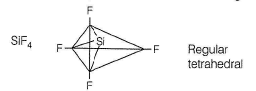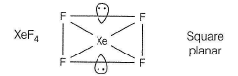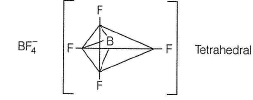QUESTION: 20

The decreasing values of bond angles from NH3 (107°) to SbH3 (91°) down group-15 of the periodic table is due to

(AIEEE 2006)

Solution: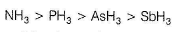As the electronegativity of central atom increases, bonded electron polarise towards central atom more, so repulsion increases and bond angle increases.

QUESTION: 21

Which of the following molecules/ions does not contain unpaired electrons?

Solution:

Molecular orbital configuration of given molecules are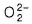(Total number of electrons = 18)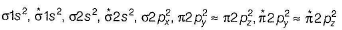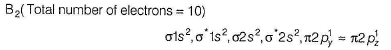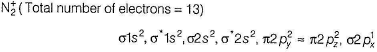Thus,does not contain any unpaired electron.

QUESTION: 22

Lattice energy of an ionic compound depends upon

(AIEEE 2005)

Solution:

Greater the charge and smaller the radius, larger the polarising power and thus, greater the covalent nature. This leads to increase in lattice energy.

QUESTION: 23

The molecular shapes of SF4 ,CF4 and XeF4 are

(AIEEE 2005)

Solution: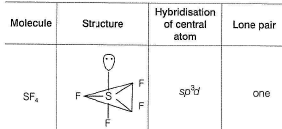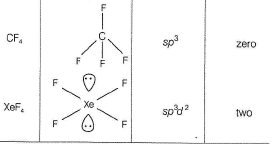QUESTION: 24

Which one of the following species is diamagnetic in nature ?

(AIEEE 2005)

Solution:

A species is said to be diamagnetic, if it has ail paired electrons.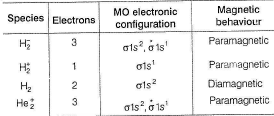QUESTION: 25

The maximum number of 90° angles between bond pair-bond pair of electrons is observed in

(AIEEE 2004)

Solution:

sp3d2 hybridisation has octahedral structure such that four hybrid orbitals are at 90° wrt each other and others two at 90° with first four.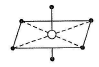QUESTION: 26

Which one of the following has the regular tetrahedral structure ?

(Atomic number : B = 5, S = 16, Ni = 28, Xe = 54)

(AIEEE 2004)

Solution:

Tetrahedral structure is associated with sp3 hybridised central atom without any lone pair. The structure of all the compounds given are as follows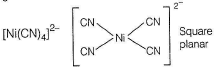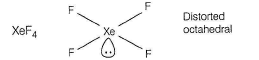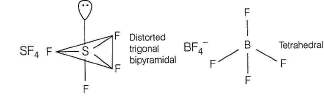QUESTION: 27

The correct order of bond angles (smallest first) in H2S, NH3, BF3 and SiH4 is

Solution: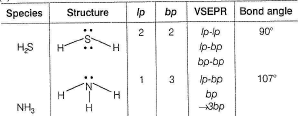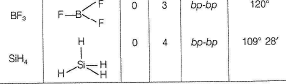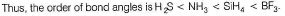QUESTION: 28

The states of hybridization of boron and oxygen atoms in boric acid (H3BO3) are respectively

(AIEEE 2004)

Solution: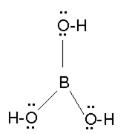Boron has three bonds thus sp2 hybridised. Each oxygen has two lone pairs hence sp3 hybridised.

QUESTION: 29

The bond order in NO is 2.5 while that in NO+ is 3. Which of the following statements is true for these two species?

(AIEEE 2004)

Solution:

Bond length is inversely proportional to bond order. Bond order in NO+ = 3 and NO = 2.5.
Thus, bond length in NO > NO+

QUESTION: 30

The pair of species having identical shapes for molecules of both species, is

(AIEEE 2003)

Solution:

XeF2 contains sp3d hybridised Xe atom with three lone pairs of electrons. Fluorine atoms occupy axial positions while three lone pairs of elelctrons occupy equatorial positions in trigonal bipyramid to minimise repulsion.
Since, lone pair of electrons do not considered for the shape of the molecule, thus, shape of XeF2 comes out to be linear. While C- atom of CO2 is sp hybridised and thus, possesses a linear shap.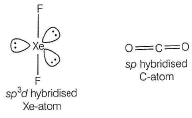QUESTION: 31

Which one of the following compounds has the smallest bond angle?

(AIEEE 2003)

Solution: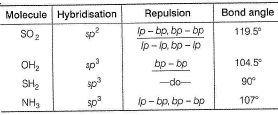QUESTION: 32

Which one of the following pairs of molecules will have permanent dipole moments for both m em bers?

(AIEEE 2003)

Solution:

Both NO2 and O3 have angular geometry, thus possess permanent dipole moment. While SiF4 possesses regular tetrahedral structure and CO2 possesses a linear structure. In SiF4 and CO2, the individual dipoles on the Si—F and O bonds cancel and the molecules have no diple moment.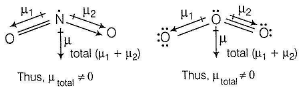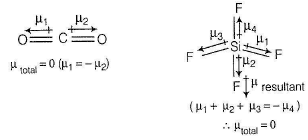QUESTION: 33

Select the correct statement.

(AIEEE 2002)

Solution: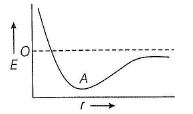As repulsive forces decrease, bond formation starts. At point A, minimum energy is attained and molecule is stable.

QUESTION: 34

The number of lone pairs on Xe in XeF2, XeF4 and XeF6 respectively, are

(AIEEE 2002)

Solution: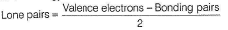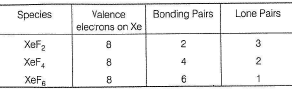QUESTION: 35

Bond angle of 109°28' is found in

Solution:

109°28 it means pure regular tetrahedral geometry.
(a) NH3, sp3, pyramidal (107°) (b) H2O. sp3, bent (104.5°).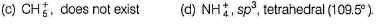QUESTION: 36

Hybridisation of the underlined under lined atom changes in

(AIEEE 2002)

Solution: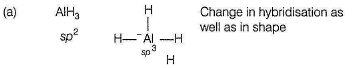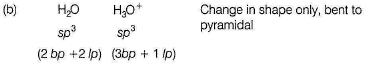QUESTION: 37

Increasing order of bond strength of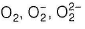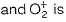(AIEEE 2002)

Solution:

Higher the bond order, greater is the bond strength.